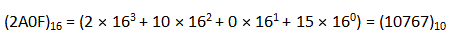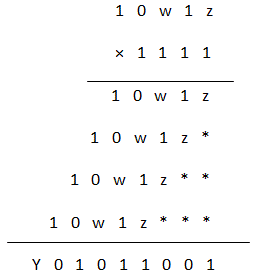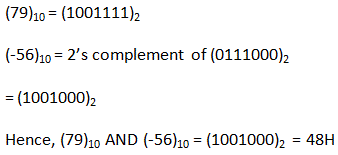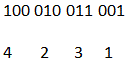# MCQs on Digital Electronics

##### Page 32 of 35. Go to page 1 2 3 4 5 6 7 8 9 10 11 12 13 14 15 16 17 18 19 20 21 22 23 24 25 26 27 28 29 30 31 32 33 34 35
01․ The decimal equivalent of hexadecimal number of 2A0F is
17670
17607
17067
1076702․ The binary equivalent of hexadecimal number 4F2D is
0101 1111 0010 1100
0100 1111 0010 1100
0100 1110 0010 1101
0100 1111 0010 1101

4→0100 F→1111 2→0010 D→1101 Hence, (4F2D)16 = (0100 1111 0010 1101)2

03․ To get an excess-3 code from BCD code
0011 is subtracted
0110 is subtracted

As name implies in excess-3 representation excess number is 3. So to get excess-3 code from BCD code we have to add 0011.

04․ Consider the following multiplication: (10w1z) × (15)10 = (y01011001)2 Which one of the following gives appropriate values of w,y and z?
w = 0,y = 0,z = 1
w = 0,y = 1,z = 1
w = 1,y = 1,z = 1
w = 1,y = 1,z = 0By comparing we get Z = 1, w = 1, y = 1

05․ Which of the following notations have two representations of zero? (I) 1’s complement with radix of number being 2 (II) 7’s complement with radix of number being 8 (III) 9’s complement with radix of number being 10 (IV) 10’s complement with radix of number being 10 Select the correct answer using the codes given below:
(I), (II) & (IV)
(I) & (III)
(II), (III) & (IV)
(I), (II) & (III)

Odd’s complement like 1’s,7’s,9’s have two representation of zero.

06․ AND operation of (79)10 & (-56)10 is
50H
48H
42H
08H07․ Statement(A): 2’s complement arithmetic is preferred in digital computers. Statement(R):The Hardware required to obtain the 2’s complement of a number is simple.
Both A & R are true and R is the correct explanation of A
Both A & R are true but R is not the correct explanation of A
A is true but R is false
A is false but R is true

All modern computers operates based on 2’s complement as hardware is faster and simpler.

08․ A new Binary Coded Pantry (BCP) number system is proposed in which every digit of a base-5 number is represented by its corresponding 3-bit binary code. For example, the base-5 number 24 will be represented by its BCP code 010100. In this numbering system,the BCP code 100010011001 corresponds to the following number in base-5 system
423
1324
2201
423109․ Which one of the following is an invalid state in 8-4-2-1 binary coded decimal counter
1000
1001
0011
1100

1100(>9) is the invalid BCD representation.

10․ The number of 1 in 8-bit representation of 127 in 2’s complement form is m and that in 1,s complement form is n. What is the value of m:n?
1:2
1:1
2:1
2:3

127→01111111 1’s complement → 10000000(n) 2’s complement → 10000001(m) Hence, m:n = 2:1

<<<3031323334>>>